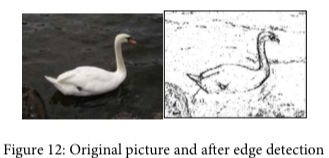function:RenderSidePart pageleftbodycaption pageleftbody sidenote Main.PictureLabActivity9-SideNote Main.SideNote Site.SideNote

# Simple edge detection

Detecting edges is a common image processing problem. For example, digital cameras often feature face detection. Some robotic competitions require the robots to find a ball using a digital camera, so the robot needs to be able to “see” a ball. One way to look for an edge in a picture is to compare the color at the current pixel with the pixel in the next column to the right. If the colors differ by more than some specified amount, this indicates that an edge has been detected and the current pixel color should be set to black. Otherwise, the current pixel is not part of an edge and its color should be set to white (Figure 12). How do you calculate the difference between two colors? The formula for the difference between two points (x1,y1) and (x2,y2) is the square root of ((x2 - x1)2 + (y2 - y1)2 ). The difference between two colors (red1,green1,blue1) and (red2,green2,blue2) is the square root of ((red2 - red1)^2 + (green2 - green1)^2 + (blue2 - blue1)^2). The colorDistance method in the Pixel class uses this calculation to return the difference between the current pixel color and a passed color.The following method implements this simple algorithm. Notice that the nested for loop stops earlier than when it reaches the number of columns. That is because in the nested loop the current color is compared to the color at the pixel in the next column. If the loop continued to the last column this would cause an out-of-bounds error.

 public void edgeDetection(int edgeDist)
{
Pixel leftPixel = null;
Pixel rightPixel = null;
Pixel[][] pixels = this.getPixels2D();
Color rightColor = null;
for (int row = 0; row < pixels.length; row++)
{
for (int col = 0;
col < pixels.length-1; col++)
{
leftPixel = pixels[row][col];
rightPixel = pixels[row][col+1];
rightColor = rightPixel.getColor();
if (leftPixel.colorDistance(rightColor) >
edgeDist)
leftPixel.setColor(Color.BLACK);
else
leftPixel.setColor(Color.WHITE);
}
}
}


You can test this with the testEdgeDetection method in PictureTester.

# Exercises

1. Notice that the current edge detection method works best when there are big color changes from left to right but not when the changes are from top to bottom. Add another loop that compares the current pixel with the one below and sets the current pixel color to black as well when the color distance is greater than the specified edge distance.
2. Work in your group to come up with another algorithm for edge detection.